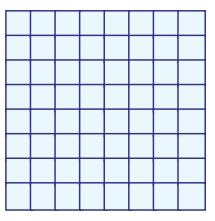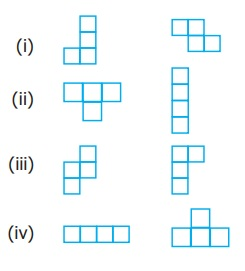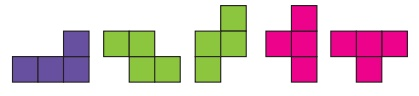Home | | Maths 7th Std | Exercise 6.1 (Tetromino)

# Exercise 6.1 (Tetromino)

7th Maths : Term 1 Unit 6 : Information Processing : Tetromino : Exercise 6.1 : Text Book Back Exercises Questions with Answers, Solution

Exercise 6.1

1. A tetromino is a shape obtained by 4 squares together.

2. Draw a tetromino which passes symmetry ……………..

Answer:3. Complete the table4. Shade the figure completely, by using five Tetromino shapes only once.5. Using the given Tetromino shaded in two different ways (), fill the grid in such a way that, no two adjacent boxes have the same colour.(more possible ways)

6. Match the tetrominoes of same type.7. Using the given tetrominoes with numbers, complete the 4 × 4 magic square .(more possible ways)

Exercise 6.1

1. 4

2.3.4.5.6.7.(more possible ways)

Tags : Questions with Answers, Solution | Information Processing | Term 1 Chapter 6 | 7th Maths , 7th Maths : Term 1 Unit 6 : Information Processing
Study Material, Lecturing Notes, Assignment, Reference, Wiki description explanation, brief detail
7th Maths : Term 1 Unit 6 : Information Processing : Exercise 6.1 (Tetromino) | Questions with Answers, Solution | Information Processing | Term 1 Chapter 6 | 7th Maths